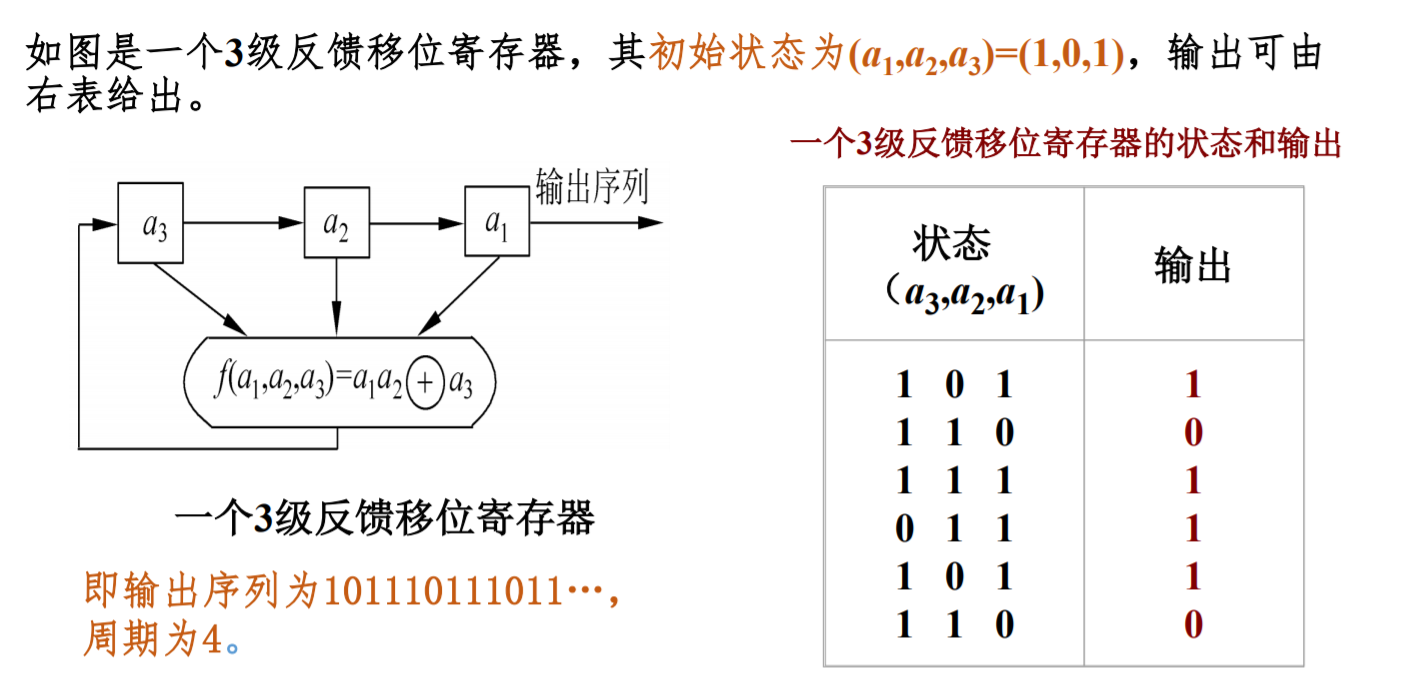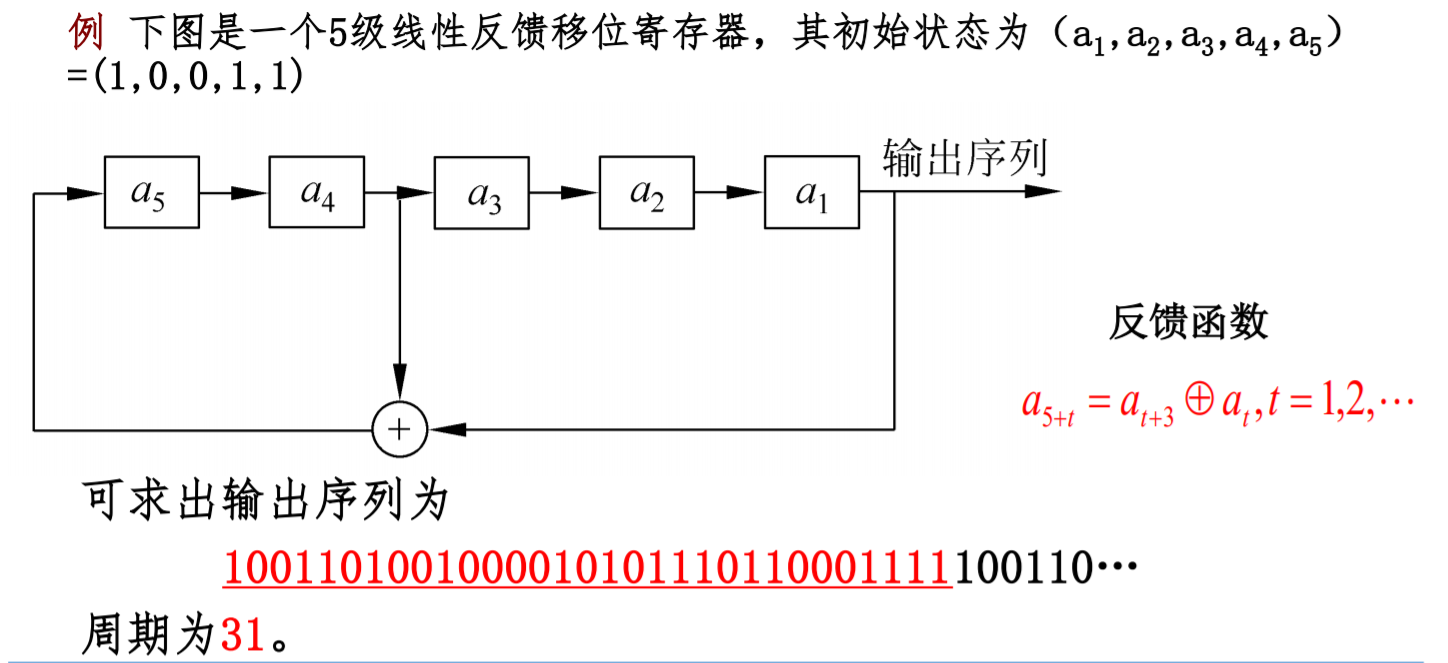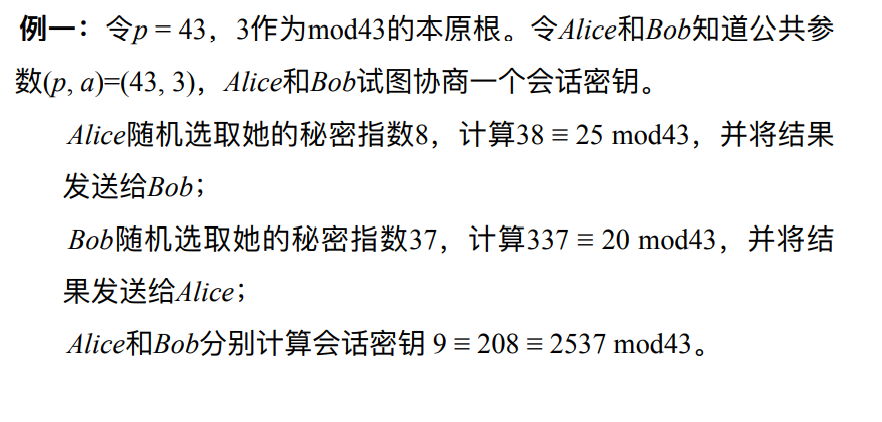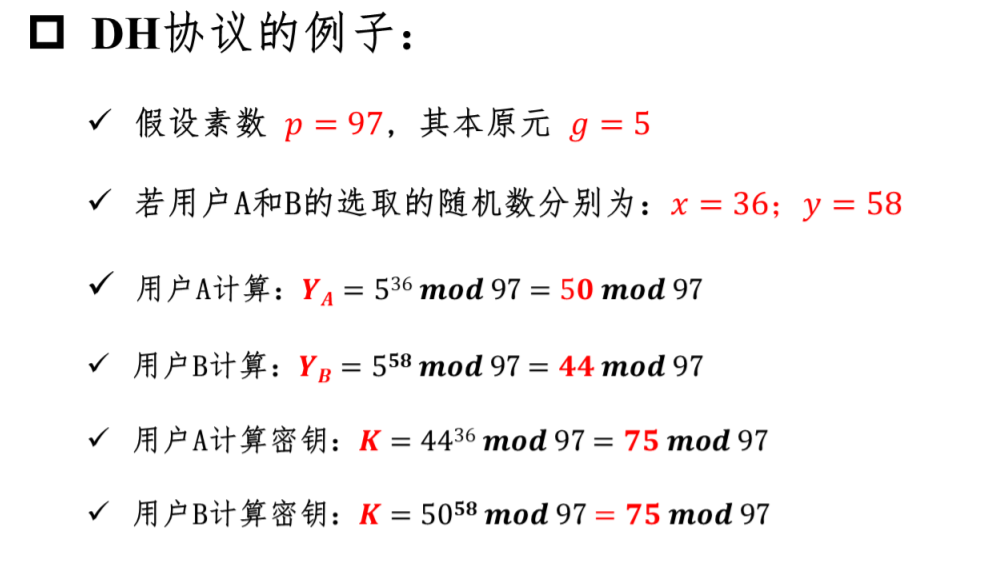密码学初探-基于RUST的密码系统与算法简析

Last update：2 months ago

Introduction

• 单表替换加密（Monoalphabetic Cipher）
• 多表替换加密（Polyalphabetic Cipher）
• 奇奇怪怪的加密方式

• 对称加密（Symmetric Cryptography），以 DES，AES，RC4 为代表。
• 非对称加密（Asymmetric Cryptography），以 RSA，ElGamal，椭圆曲线加密为代表。
• 哈希函数（Hash Function），以 MD5，SHA-1，SHA-512 等为代表。
• 数字签名（Digital Signature），以 RSA 签名，ElGamal 签名，DSA 签名为代表。

• 分组密码（Block Cipher），又称为块密码。
• 序列密码（Stream Cipher），又称为流密码。

• 机密性（Confidentiality）
• 完整性（Integrity）
• 可用性（Availability）
• 认证性（Authentication）
• 不可否认性（Non-repudiation）

仿射密码

原理

• $$x$$ 表示明文按照某种编码得到的数字
• $$a$$$$m$$ 互质
• $$m$$ 是编码系统中字母的数目。

x 0 5 5 8 13 4 2 8 15 7 4 17
$$y=5x+8$$ 8 33 33 48 73 28 18 48 83 43 28 93
$$y\mod26$$ 8 7 7 22 21 2 18 22 5 17 2 15

$$y$$ 8 7 7 22 21 2 18 22 5 17 2 15
$$x=21(y-8)$$ 0 -21 -21 294 273 -126 210 294 -63 189 -126 147
$$x\mod26$$ 0 5 5 8 13 4 2 8 15 7 4 17

破解

$\phi(26)=\phi(2) \times \phi(13) = 12$

$12 \times 26 = 312$

$y_1=(ax_1+b)\pmod{26} \\ y_2=(ax_2+b)\pmod{26}$

$y_1-y_2=a(x_1-x_2)\pmod{26}$

流密码

• 流密码的密钥长度会与明文的长度相同。
• 流密码的密钥派生自一个较短的密钥，派生算法通常为一个伪随机数生成算法。

反馈移位寄存器

• $$a_0$$$$a_1$$，…，$$a_{n-1}$$ 为初态。
• F 为反馈函数或者反馈逻辑。如果 F 为线性函数，那么我们称其为线性反馈移位寄存器（LFSR），否则我们称其为非线性反馈移位寄存器（NFSR）。
• $$a_{i+n}=F(a_i,a_{i+1},...,a_{i+n-1})$$

$$(a_i,a_{i+1},...,a_{i+n-1}) \rightarrow (a_{i+1},...,a_{i+n-1},a_{i+n})$$ . 对于一个序列来说，我们一般定义其生成函数为其序列对应的幂级数的和。

线性反馈移位寄存器 - LFSR

介绍

$$a_{i+n}=\sum\limits_{j=1}^{n}c_ja_{i+n-j}$$

\begin{align*} &\left[ a_{i+1},a_{i+2},a_{i+3}, ...,a_{i+n} \right]\\\ \\\ =&\left[ a_{i},a_{i+1},a_{i+2}, ...,a_{i+n-1} \right]\left[ \begin{matrix} 0 & 0 & \cdots & 0 & c_n \\\ 1 & 0 & \cdots & 0 & c_{n-1} \\\ 0 & 1 & \cdots & 0 & c_{n-2} \\\ \vdots & \vdots & \ddots & \vdots \\\ 0 & 0 & \cdots & 1 & c_1 \\\ \end{matrix} \right] \\\ \\\ =&\left[ a_{0},a_{1},a_{2}, ...,a_{n-1} \right]\left[ \begin{matrix} 0 & 0 & \cdots & 0 & c_n \\\ 1 & 0 & \cdots & 0 & c_{n-1} \\\ 0 & 1 & \cdots & 0 & c_{n-2} \\\ \vdots & \vdots & \ddots & \vdots \\\ 0 & 0 & \cdots & 1 & c_1 \\\ \end{matrix} \right]^{i+1} \end{align*}

$$f(x)=x^n-\sum\limits_{i=1}^{n}c_ix^{n-i}$$

$$\overline f(x)=x^nf(\frac{1}{x})=1-\sum\limits_{i=1}^{n}c_ix^{i}$$

样例特征多项式与生成函数

$$A(x)=\frac{p(x)}{\overline f(x)}$$

特殊性质

• 将两个序列累加得到新的序列的周期为这两个序列的周期的和。
• 序列是 n 级 m 序列，当且仅当序列的极小多项式是 n 次本原多项式。

B-M 算法

• 密钥生成角度，一般我们希望使用级数尽可能低的 LFSR 来生成周期大，随机性好的序列。
• 密码分析角度，给定一个长度为 n 的序列 a，如何构造一个级数尽可能小的 LFSR 来生成它。其实这就是 B-M 算法的来源。

• 若 s 为一个全零序列，则线性复杂度为0。
• 若没有 LFSR 能生成 s，则线性复杂度为无穷。
• 否则，s 的线性复杂度为生成 L(s) 的最小级的 LFSR。

BM 算法的要求我们需要知道长度为 2n 的序列。其复杂度

• 时间复杂度：O(n^2) 次比特操作
• 空间复杂度：O(n) 比特。

$$S_1=(a_1,...,a_n)$$

$$S_2=(a_2,...,a_{n+1})$$

....

$$S_{n+1}=(a_{n+1},...,a_{2n})$$

$$S_{n+1}=(c_n,...,c_1)X$$

$$(c_n,...,c_1)=S_{n+1}X^{-1}$$

非线性反馈移位寄存器

介绍

• 非线性组合生成器，对多个 LFSR 的输出使用一个非线性组合函数
• 非线性滤波生成器，对一个 LFSR 的内容使用一个非线性组合函数
• 钟控生成器，使用一个（或多个）LFSR 的输出来控制另一个（或多个）LFSR 的时钟

RC4

基本介绍

RSA 由 Ron Rivest 设计，加解密使用相同的密钥，因此也属于对称加密算法。它是面向字节的流密码，密钥长度可变，非常简单，但也很有效果。RC4 算法曾广泛应用于 SSL/TLS 协议和 WEP/WPA 协议，但由于RC4算法存在弱点，2015年2月所发布的 RFC 7465 规定禁止在TLS中使用RC4加密算法。

基本流程

RC4 主要包含三个流程

• 初始化 S 和 T 数组。
• 初始化置换 S。
• 生成密钥流。

块加密

概述

• IDEA 加密
• DES 加密
• AES 加密

• 分组加密模式，即明文分组加密的方式。

工作模式

1. 电码本(ECB)模式
2. 密码分组链接(CBC)模式
3. 密码反馈(CFB)模式
4. 输出反馈(OFB)模式
5. 计数器(CTR)模式

• S 盒
• 乘法

• 线性变换
• 置换
• 移位，循环移位

常见加解密结构

• 迭代结构，这是因为迭代结构便于设计与实现，同时方便安全性评估。

迭代结构

• 密钥置换
• 轮加密函数
• 轮解密函数

轮函数

• Feistel Network，由 Horst Feistel 发明，DES 设计者之一。
• DES
• Substitution-Permutation Network(SPN)
• AES
• 其他方案

DES

基本介绍

Data Encryption Standard(DES)，数据加密标准，是典型的块加密，其基本信息如下

• 输入 64 位。
• 输出 64 位。
• 密钥 64 位，使用 64 位密钥中的 56 位，剩余的 8 位要么丢弃，要么作为奇偶校验位。
• Feistel 迭代结构
• 明文经过 16 轮迭代得到密文。
• 密文经过类似的 16 轮迭代得到明文。

基本流程

加密

$$L_{i+1}=R_i$$

$$R_{i+1}=L_i\oplus F(R_i,K_i)$$

解密

$$R_i=L_{i+1}$$

$$L_i=R_{i+1}\oplus F(L_{i+1},K_i)$$

核心部件

DES 中的核心部件主要包括（这里只给出加密过程的）

• 初始置换
• F 函数
• E 扩展函数
• S 盒，设计标准未给出。
• P 置换
• 最后置换

衍生

• 双重 DES
• 三种 DES

双重 DES

$$C=E_{k2}(E_{k1}(P))$$

$$I={E_{k1}(P)}$$

$$J=D_{k2}(C)$$

三重 DES

$$C=E_{k3}(D_{k2}(E_{k1}(P)))$$

$$P=D_{k1}(E_{k2}(D_{k3}(C)))$$

• 3 个不同的密钥，k1，k2，k3 互相独立，一共 168 比特。
• 2 个不同的密钥，k1 与 k2 独立，k3=k1，112 比特。

• 差分攻击
• 线性攻击

AES

基本介绍

Advanced Encryption Standard（AES），高级加密标准，是典型的块加密，被设计来取代 DES，由 Joan Daemen 和 Vincent Rijmen 所设计。其基本信息如下

• 输入：128 比特。
• 输出：128 比特。
• SPN 网络结构。

128 10
192 12
256 14

基本流程

加解密过程

• 字节替换，SubBytes
• 行移位，ShiftRows
• 列混淆，MixColumns

等价解密算法

• 交换逆向行移位和逆向字节代替并不影响结果。
• 交换轮密钥加和逆向列混淆并不影响结果，关键在于
• 首先可以把异或看成域上的多项式加法
• 然后多项式中乘法对加法具有分配率。

• 积分攻击

非对称加密

RSA

RSA 算法的可靠性由极大整数因数分解的难度决定。换言之，对一极大整数做因数分解愈困难，RSA 算法愈可靠。假如有人找到一种快速因数分解的算法的话，那么用 RSA 加密的信息的可靠性就肯定会极度下降。但找到这样的算法的可能性是非常小的。如今，只有短的 RSA 密钥才可能被强力方式解破。到 2020 年为止，还没有任何可靠的攻击 RSA 算法的方式。

基本原理

公钥与私钥的产生

1. 随机选择两个不同大质数 $$p$$$$q$$，计算 $$N = p \times q$$
2. 根据欧拉函数，求得 $$\varphi (N)=\varphi (p)\varphi (q)=(p-1)(q-1)$$
3. 选择一个小于 $$\varphi (N)$$ 的整数 $$e$$，使 $$e$$$$\varphi (N)$$ 互质。并求得 $$e$$ 关于 $$\varphi (N)$$ 的模反元素，命名为 $$d$$，有 $$ed\equiv 1 \pmod {\varphi (N)}$$
4. $$p​$$$$q​$$ 的记录销毁

消息加密

$m^{e}\equiv c\pmod N$

消息解密

$c^{d}\equiv m\pmod N$

正确性证明

$m^{k\phi(N)+1} \equiv m \bmod N$

$m=xp$

$m^{\phi(q)} \equiv 1 \bmod q$

$m^{k\phi(N)}=m^{k(p-1)(q-1)}=(m^{\phi(q)})^{k(p-1)} \equiv 1 \bmod q$

$m^{k\phi(N)+1}=m+uqm$

$m^{k\phi(N)+1}=m+uqxp=m+uxN$

样例

例1

计算公钥和私钥
1. p = 13 , q = 5

• N = pq = 65
• r = (p-1)(q-1) = (13-1)(5-1) = 48
2. 计算模反元素 r=48，选择e=5，得到二元一次方程：5d-48k=1 , 获得一组解：d=29，k=3

3. 因此，公钥是 (N, e) = (65, 5)，私钥是 (N, d) = (65, 29)。

加密信息
1. 明文：m=3

2. 计算: $c ^{5}$

3. 因此：3被加密为48

解密信息
1. 密文：c=48

2. 计算：$n ^{29}$

3. 因此：48被解密为3

密码协议

Diffie-Hellman 密钥交换

• 密钥交换是实现安全通信的基础
• 商用加密算法AES和DES需要在安全通信之前，实现通信双方的密钥共享。
• 密钥交换的方法：
• 基于RSA的密钥交换；
• 基于KDC技术 (Key Distributed Center，密钥分发中心)；
• Diffie-Hellman密钥交换（简称：DH算法）；
• 基于物理层的密钥交换。

DH算法是不安全信道下实现安全密钥共享的一种方法，由 W. Diffie 和 M.Hellman 在1976年提出的第一个公开的公钥密码算法